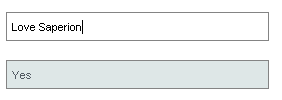Search

# How to get “IF” Statements going in SAPERION

## Aim of this article

SAPERION is not very forthcoming when it comes to documentation relating to formulas. Well, most of the documentation can be summarized as, well, vague. So I have endeavored to create a conditional statement in a text field. I will not expand on the various formulas I tried, but will give you a summary of test formulas that you can also try.

## Setup the test

Let us start with any arbitrary 2 field mask

Search, a variable fieldDescription, a DDC fieldYou should now have something like thisNow let us assign a default value to the first field, the value is =”Love Saperion”. This will be our test stringNow we will need a formula to start testing. Enter the description field. We will create a formula that tests whether there are any spaces in the @Search variable field.

1. To do this we will use the Pos function

Use this formula in the Default value box:

Pos (” “, @Search) > 0 ? “Yes” : “No”Test the mask

Hit the test button1. Is there a space? Seems like the formula is working.1. Now remove the ” Saperion” form the search field and click in another field to recalculate.1. It seems to work.

## But what has happened here?

Let us expand the formula to understand how the formula can resemble something like =if(1=2,True,False)

1. Let us start with the formula

Pos (” “, @Search) > 0    ?     “Yes”         :     “No”

< The logic test >        ?    <True>     :    <False>

1. Now let us translate it to something perhaps more Excel-ish

IF(Find(” “,@Search)    , “Yes”         , “No”)

< The logic test >        ,    <True>     ,    <False>

## Conclusion

It is quite simple, just take out some brackets and the formula makes sense. The biggest problem is testing and troubleshooting for find the right formula to work in SAPERION.

## Sample Formulas

Give theses a go as well:

SysDate:

SysTime:

MachineName

DBName

='”‘+ (Pos (” “, @Search) > 0 ? (Copy (@Search, 0, Pos (” “, @Search)) + ‘*” AND “‘ + Copy (@Search, Pos (” “, @Search)+1, Length(@Search) – Pos (” “, @Search))) : (@Search) )+’*”‘

## Further Reading

0 views0 comments

### Recent Posts

See All

#### Sometimes all SAPERION needs is an open port.

When trying to connect multiple SAPERION servers in a farm most of the backed stuff gets handled by SAPERION, which is quite nice. But there are some items that you never get prompted for, enter the c